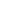### الصفحاتم4 Design مرحلة رابعة

# Wear Experiment مختبر التصميم

Experiment No. 6

Name: Wear

Aim: To study the performance of the brakes & clutches through the measurement of the wear that taken place in the friction disk material at different applicable pressure and running rotational speed of the brake flange.

Theory:

Wear is progressive damage to a surface caused by relative motion with respect to another substance. There are three apparent ways in which wear may be classified. One is in terms of the appearance of the wear scar. A second way is in terms of the physical mechanism that removes the material or causes the damage. The third is in terms of the conditions surrounding the wear situation. Most wear mechanisms are built around concepts such as brittle fracture, plastic deformation, fatigue, and cohesive and adhesive failures in bonded structures.

A study of the friction disc will be studied in this experiment through understanding the effect of the different design parameters that to be taken in consideration, like the cars clutches and wheel brakes.

The linear measure of wear w is expressed in inches or millimeters. The work done by force [ fS P A] during displacement S is [ fS P AS ] or [ fS P AV t] , where V is the sliding velocity and t is time. The material volume removed due to wear is  [ w A] and is proportional to the work done, that is,[ w A fS P AV t ], or the linear wear is then expressed as:w = K P V t = f 1 f 2 K P V t = const. * P * N

Where;  P is expressed in psi, V in fpm , and  t in hours. This makes the units of  K in3· min/(lbf · ft · h) [US  units]. While for K are cm3.min/(kgf · m · h), where 1 kgf = 9.806 N [SI units]. The modifying factor f 1 depending on motion type, load, and speed and an environment, while the factor f 2 to account for temperature and cleanliness conditions.

The values of [ K,  f 1  , & f 2 ] are given in the below table;

Circular (Button or Puck) Pad Caliper BrakeThe above figure displays the pad geometry. Numerical integration is necessary to analyze this brake since the boundaries are difficult to handle in closed form. The attached table gives the parameters for this brake as determined by Fazekas.

The effective radius is given by re = δ e

The actuating force is given by:   F = π R2 pav

The torque is given by:  T = f F re

The attached figures show the front wheel disk brakes of the car and the distribution pressure on the disk brake material.

Procedure:

1-    Measure the weight of the friction pads before starting the test.

2-    Fix the friction pads in the machine.

3-    Adjust the belts speed mechanism to get a certain value of speed, and adjust the hydraulic pressure to 2 bars.

4-    Run the machine for 2 minutes, and ensure that the friction pads are in contact with the steel disc during the test.

5-    Switch off the testing machine and take the friction pads out and measure its weight and calculate the wear percentage.

6-    Re-fix the friction pads and increase the pressure to 4 bar while the speed is hold as before and retest the pads for 2 minutes also.

7-    Repeat step [ 5 ], then repeat step [ 6 ] with pressure new pressure  bar and repeat step [ 5 ].

8-      Change the speed to two higher values and repeat steps [ 4,5,& 6 ] for each of these speeds.

% wear = [( Original weight – Final weight ) / Original weight] * 100

% Wear Test Table for the Friction Pad

 Case N1 = N2 = N3 = P = 2 bar P = 4 bar P = 6 bar P = 2 bar P = 4 bar P = 6 bar P = 2 bar P = 4 bar P = 6 bar Right Pad Left Pad

Calculation & Discussion:

1-    Calculate the wear percentage for each studied case of pressure or speed.

2-    Draw the wear percentage with the pressure change / and the speed change.

3-    Discuss the factors that affecting the wear rate.

4-    What are the factors that limiting the selection of the friction pads for the car wheels.

5-    Give with sketches two application examples for the braking system.مهندس ميكانيك
هدفي هو التطوير من ذاتي ثم الاستفادة ثم تطوير غيري